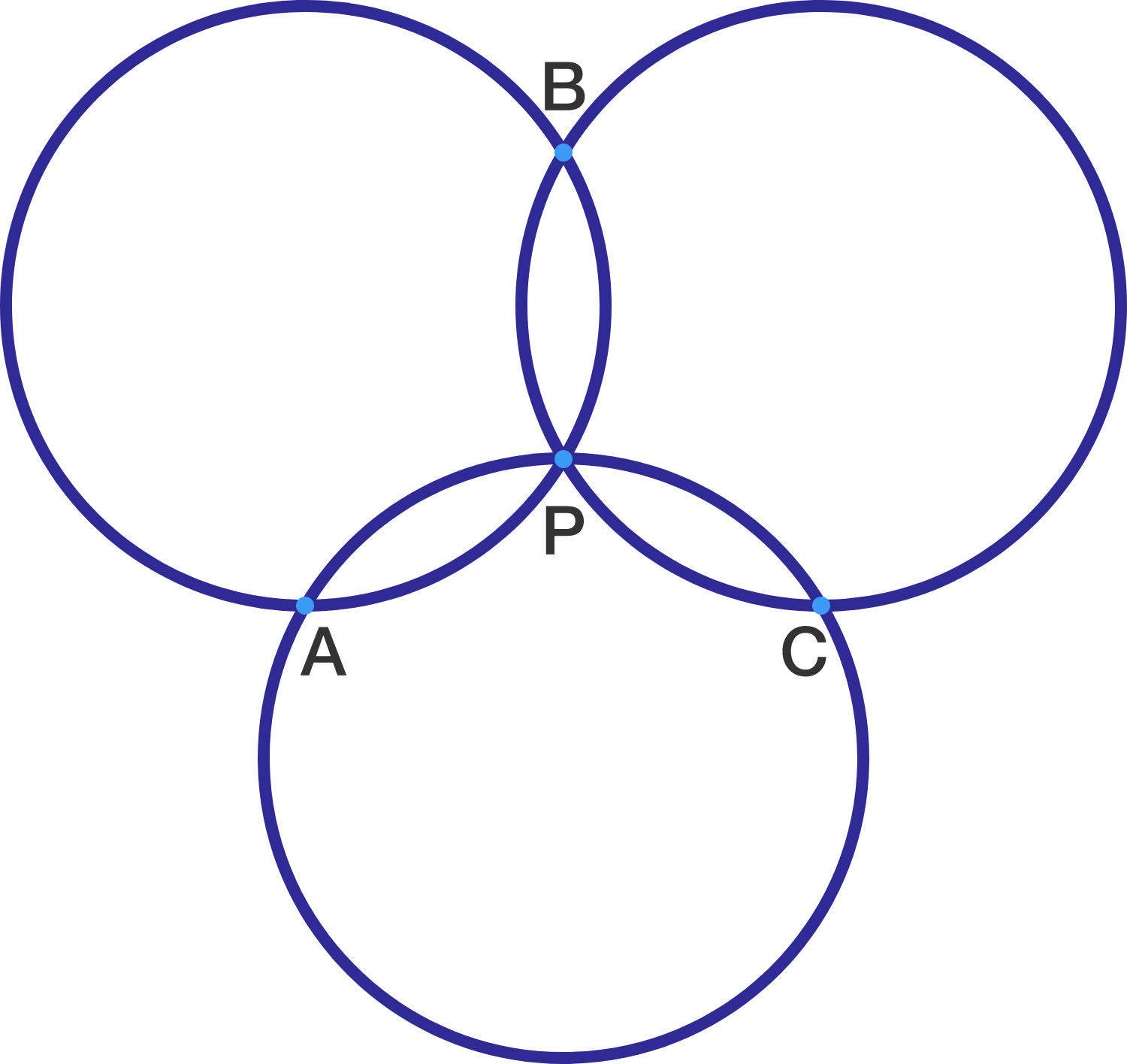# Angle Chasing Helps, What About Dilation?

Geometry Level 2Three circles of equal radii all intersect at a single point $P$. Let the other intersections be $A$, $B$ and $C$. Which of the following must be true?

×

Problem Loading...

Note Loading...

Set Loading...